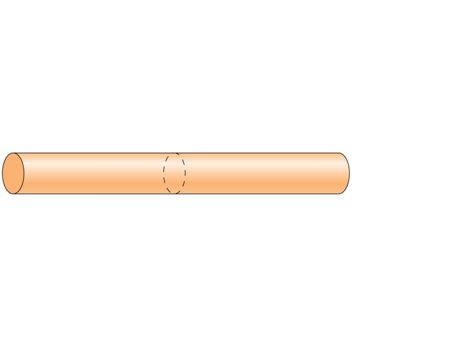# Problem: Every minute, 120 C of charge flow through this cross section of the wire. The wire's current is:A) 120AB) 240AC) 2AD) Some Other valueE) 60A

###### FREE Expert Solution

Current:

$\overline{){\mathbf{i}}{\mathbf{=}}\frac{\mathbf{d}\mathbf{q}}{\mathbf{d}\mathbf{t}}}$###### Problem DetailsEvery minute, 120 C of charge flow through this cross section of the wire. The wire's current is:

A) 120A

B) 240A

C) 2A

D) Some Other value

E) 60A